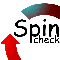# Spinroot

A forum for Spin users

You are not logged in.

## #1 2011-07-30 23:30:32

Chengxiu
Member
Registered: 2011-07-28
Posts: 19

### about concurrency issue of two processes

Dear all,

I am trying to do the following program: two processes, named Robot1 and Robot2, they have four global variables, robot1x, robot1y, robot2x, robot2y.
proctype Robot1 assigns robot1x and robot1y, and assigns its internal variable connected1 by comparisons of robot1x, robot1y, robot2x, robot2y.
proctype Robot2 assigns robot2x and robot2y, and assigns its internal variable connected2 by comparisons of robot1x, robot1y, robot2x, robot2y.

the Program is as follows:

--------------------------------------------------------------------------

int robot1x;
int robot1y;
int robot2x;
int robot2y;
mtype = {n, s, e, w};

proctype Robot1()
{
int robot1Loc = 0;
int range =1;
int lenmax = 5;
int abs12x;
int abs12y;
bool connected1 = 0;

robot1x = robot1Loc / 10;
robot1y = robot1Loc % 10;

if
::(robot2x >= robot1x) -> abs12x = robot2x - robot1x;
::(robot2x < robot1x) -> abs12x = robot1x - robot2x;
fi

if
::(robot2y >= robot1y) -> abs12y = robot2y - robot1y;
::(robot2y < robot1y) -> abs12y = robot1y - robot2y;
fi

connected1 = ((abs12x <= range) || (lenmax - abs12x) <= range)) && ((abs12y <= range) || (lenmax - abs12y) <= range));

}

proctype Robot2()
{
int robot2Loc = 11;
int range =1;
int lenmax = 5;
int abs12x;
int abs12y;
bool connected2 = 0;

robot2x = robot2Loc / 10;
robot2y = robot2Loc % 10;

if
::(robot2x >= robot1x) -> abs12x = robot2x - robot1x;
::(robot2x < robot1x) -> abs12x = robot1x - robot2x;
fi

if
::(robot2y >= robot1y) -> abs12y = robot2y - robot1y;
::(robot2y < robot1y) -> abs12y = robot1y - robot2y;
fi

connected2 = ((abs12x <= range) || (lenmax - abs12x) <= range)) && ((abs12y <= range) || (lenmax - abs12y) <= range));

}

init { atomic {run Robot1(); run Robot2()} }

-----------------------------------------------------------------------------

when I run the simulation, I hope the result shows both connected1, connected2 = 1.
but the system reports there is syntax errors in process Robot1 at:

if
::(robot2x >= robot1x) -> abs12x = robot2x - robot1x;
::(robot2x < robot1x) -> abs12x = robot1x - robot2x;
fi

I think it is because the system does not know the value of robot2x, which will be later
assigned in process Robot2(), How to solve this problem?

Cheers,

Chengxiu

Offline

## #2 2011-07-31 01:36:36

spinroot
forumRegistered: 2010-11-18
Posts: 691
Website

### Re: about concurrency issue of two processes

there's a missing semi-colon (';') after the two 'if' statements (lines 22 and 27)
and similarly in the other proctype
all statements (including compound statements) must be separated by semi-colons in Promela

Offline